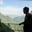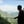Trusted answers to developer questions
Trusted Answers to Developer Questions

Related Tags

ai
propositional
communitycreator

# What is first-order logic in Artificial Intelligence?AKASH BAJWA

Grokking Modern System Design Interview for Engineers & Managers

Ace your System Design Interview and take your career to the next level. Learn to handle the design of applications like Netflix, Quora, Facebook, Uber, and many more in a 45-min interview. Learn the RESHADED framework for architecting web-scale applications by determining requirements, constraints, and assumptions before diving into a step-by-step design process.

To understand why we should use first-order logic (FOL), we must first understand the need for it and background behind it.

### What is propositional logic?

Propositional logic (PL) is declarative, so it guides us on how to represent information in a logical form and draw conclusions.

We can only represent information as either true or false in propositional logic.

### Disadvantages of PL

• If you want to represent complicated sentences or natural language statements, PL is not sufficient.

• There is very limited expressive power in PL, so we use FOLfirst-order logic instead.

The sentence shown below cannot be represented we cannot using PL logic.

### Examples

1. I love mankind. It’s the people I can’t stand!

2. Joe Root likes football.

3. I like to eat mangos.

PL is not enough to represent the sentences above, so we require powerful logic (such as FOL).

### What is first-order logic (FOL)?

1. FOL is a mode of representation in Artificial Intelligence. It is an extension of PL.

2. FOL represents natural language statements in a concise way.

3. FOL is also called predicate logic. It is a powerful language used to develop information about an object and express the relationship between objects.

4. FOL not only assumes that does the world contains facts (like PL does), but it also assumes the following:

• Objects: A, B, people, numbers, colors, wars, theories, squares, pit, etc.

• Relations: It is unary relation such as red, round, sister of, brother of, etc.

• Function: father of, best friend, third inning of, end of, etc.

### Parts of first-order logic

FOL also has two parts:

1. Syntax
2. Semantics

### Syntax

The syntax of FOL decides which collection of symbols is a logical expression.

The basic syntactic elements of FOL are symbols. We use symbols to write statements in shorthand notation.

Basic elements of FOL
 Name Symbol Constant 1, 6, A,W,New York, Elie, Dog... Variables a, b, c, x, y, z... Predicates <, >, brother, sister, father... Equality == Function Sqrt, LessThan, Sin(θ)... Quantifier ∀, ∃ Connectives ∧, ∨, ¬, ⇒, ⇔

### Atomic and complex sentences in FOL

#### 1. Atomic Sentence

• This is a basic sentence of FOL formed from a predicate symbol followed by a parenthesis with a sequence of terms.

• We can represent atomic sentences as a predicate (value1, value2…., value n).

#### Example

1. John and Michael are colleagues → Colleagues (John, Michael)

2. German Shepherd is a dog → Dog (German Shepherd)

#### 2. Complex sentence

Complex sentences are made by combining atomic sentences using connectives.

FOL is further divided into two parts:

• Subject: the main part of the statement.

• Predicate: defined as a relation that binds two atoms together.

#### Example

1. Colleague (Oliver, Benjamin) ∧ Colleague (Benjamin, Oliver)

2. “x is an integer”

It has two parts;

• first, x is the subject.
• second, “is an integer” is called a predicate.

### Propositional logic vs. first-order logic

#### Propositional logic

Propositional logic assumes that some facts exist that can either hold or do not hold.

#### First-order-logic

The universe consists of multiple objects with certain relations among them that can either hold or do not hold.

There are other special-purpose logics that support FOL:

1. Fuzzy logic
2. Higher-order logic
3. Temporal logic
4. Probability theory

### Quantifiers and their use in FOL

Quantifiers generate quantification and specify the number of specimen in the universe.

• Quantifiers allow us to determine or identify the range and scope of the variable in a logical expression.

• There are two types of quantifiers:

1. Universal quantifier: for all, everyone, everything.

2. Existential quantifier: for some, at least one.

#### 1. Universal quantifiers

• Universal quantifiers specify that the statement within the range is true for everything or every instance of a particular thing.

• Universal quantifiers are denoted by a symbol (∀) that looks like an inverted A. In a universal quantifier, we use  →.

• If x is a variable, then ∀x can read as:

1. For all x
2. For every x
3. For each x

#### Example

Every Student Likes Educative.

#### Explanation

So, in logical notation, it can be written as:

∀x student(x) → likes(x, Educative)

This can be interpreted as: There is every x where x is a student who likes Educative.

#### 2. Existential quantifiers

• Existential quantifiers are used to express that the statement within their scope is true for at least one instance of something.

• ∃, which looks like an inverted E, is used to represent them. We always use AND or conjunction symbols.

• If x is a variable, the existential quantifier will be ∃x:

1. For some x
2. There exists an x
3. For at least one x

#### Example

Some people like Football.

#### Explanation

So, in logical notation, it can be written as:

∃x: people(x) ∧ likes Football(x)

It can be interpreted as: There are some x where x is people who like football.

### Nested quantifiers & their uses

We can use both quantifiers together, but it’s not a type of quantifier; rather, it’s an outlier category.

• Nestedinside of another set/function quantifier refers to when one quantifier is within the scope of another quantifier.

• These quantifiers can be represented using the ∃x∀x signs.

• Here are some examples to understand this type of quantifier.

1. ∃xy ∀x ∀y((x< 0) ∧ (y< 0) → (xy = 8))

This can be interpreted as: For every real number x and yℝ, if x is negative and y is also negative, implies for some values of xy must be equal to 8.

RELATED TAGS

ai
propositional
communitycreator

CONTRIBUTORAKASH BAJWA

Grokking Modern System Design Interview for Engineers & Managers

Ace your System Design Interview and take your career to the next level. Learn to handle the design of applications like Netflix, Quora, Facebook, Uber, and many more in a 45-min interview. Learn the RESHADED framework for architecting web-scale applications by determining requirements, constraints, and assumptions before diving into a step-by-step design process.

Learn in-demand tech skills in half the time